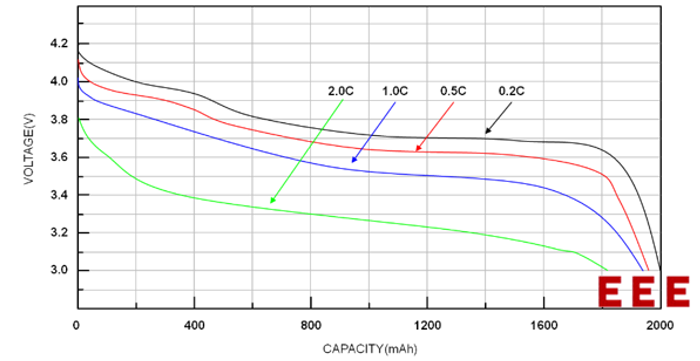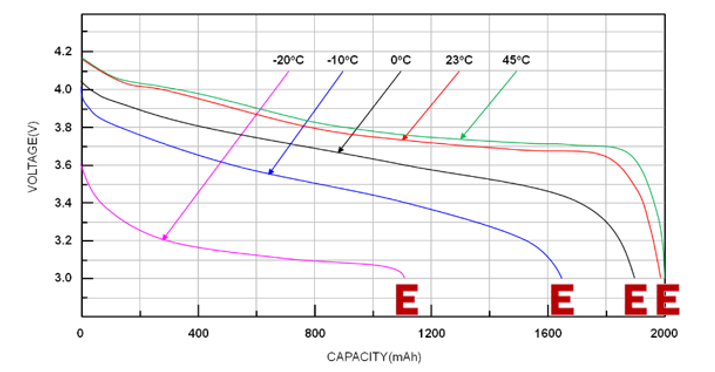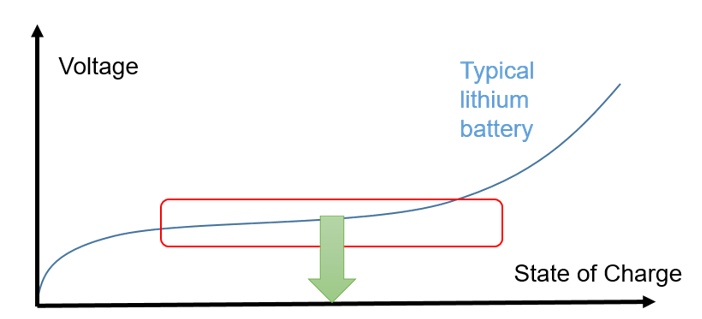# Determining Accurate State of Charge with the MPF4279x Series (Part I)Get valuable resources straight to your inbox - sent out once per month

## Introduction

With the dramatic improvement in battery performance, applications are increasingly utilizing battery packs to provide energy. In the battery management system (BMS), designers face the challenge of accurately estimating the battery’s state-of-charge (SoC), which is the level of charge a battery has in relation to its capacity.

SoC is commonly represented as a percentage between 0% and 100%. A battery is fully discharged at SoC = 0% and fully charged at SoC = 100%. SoC can help calculate the power of everyday technologies, including mobile phones, smartwatches, and electric vehicles. However, errors in estimating SoC arise due to the battery’s complex chemical characteristics.

This article is the first of a two-part series discussing SoC estimations. Part I explores the complex application of battery conditions in SoC estimation, as well one method to estimate the SoC. Part II will discuss additional methods and introduce the MPF4279x series.

## Challenges of SoC Estimation

If the battery voltage drops below a certain threshold while it discharges, the battery is considered empty. A battery is considered fully charged when is it charged with a constant voltage and a current within the specific threshold. When the battery transitions from discharging to charging, the battery’s capacity can vary due to several factors.

Due to the battery’s internal resistance, the charge and discharge rates are different, which results in different capacities. Batteries can be made of different materials with unique temperature state characteristics. Figure 1 shows the battery voltage under different discharge current magnitudes.Figure 1: Battery Capacity vs. Discharge Rate

In particular, reduced battery performance is observed at low temperatures. Figure 2 shows the battery’s capacity across a wide temperature range.Figure 2: Battery Capacity vs. Temperature

The battery’s life status characteristics also contribute to its capacity. Generally, battery life gradually decays during use. This decay can be largely attributed to the degradation of the positive and negative materials and the passivation of electrodes, which leads to a loss in effective lithium ions. Furthermore, the total power changes from beginning of life (BOL) to end of life (EOL). This means that it is important to consider the total capacity at BOL or the actual total capacity in the current lifecycle when calculating SoC.

In addition to the varying battery capacity, the SoC status of each cell affects the SoC estimate for the total battery pack. When multiple batteries are connected in series, the battery voltage can be unbalanced.

## Introduction to SoC Algorithms

There are three key methods to calculate the SoC: the open-circuit voltage (OCV), ampere-hour integration, and voltage-current hybrid methods. The first method is discussed in this article, while the remaining methods will be discussed in Part II.

## Open-Circuit Voltage (OCV) Method

The OCV method is one of the earliest battery capacity test methods based on the change between the battery’s OCV and the lithium ion concentration inside the battery. This reflects the monotonic increasing relationship between OCV and SoC.

While the OCV method is straightforward and convenient, it does not provide high accuracy, and it can only properly estimate the SoC when the battery has been at rest for a significant time. When current flows through the battery, the voltage drop generated by the battery’s internal resistance affects the accuracy of the SoC estimate. At the same time, batteries like the lithium iron phosphate battery may have a voltage plateau. When the SoC is between 30% and 80%, the relationship between the terminal voltage and SoC is a relatively straight line, which amplifies the SoC estimation error (see Figure 3).Figure 3: Relationship between Lithium Battery OCV and SoC

To address these issues, designers supplemented the OCV method by incorporating the battery’s internal resistance to correct and accurately estimate OCV. As current flows through the battery, the voltage under the load is corrected by subtracting the actual measured battery-side voltage drop (from I x R) from the OCV. The estimated SoC (V) can be calculated with Equation (1).

$$V = OCV - I \times\ R(SoC, T)$$

Where OCV is the open-circuit voltage, I is the applied current, and R is the equivalent series resistance, which depends on SoC and temperature (T). Note that in this equation, a positive current is used for discharge and a negative current is used for charge.

Using internal resistance compensation increases SoC estimation accuracy. However, the complex electrochemical properties in practical application prevent the battery voltage from immediately responding to load changes. This delay is associated with the time constant, ranging from milliseconds to thousands of seconds. The battery’s internal impedance also varies significantly under different conditions, which means an accurate SoC estimate depends on an accurate impedance estimate.

## Conclusion

This article introduced the concept of SoC estimates, as well as the challenges in obtaining an accurate SoC. We also discussed one method to estimate SoC. Part II will expand on the other two methods and explain the benefits of using the MPF4279x series to accurately measure the SoC.

### Did you find this interesting? Get valuable resources straight to your inbox - sent out once per month!

Get technical support